# R Data Types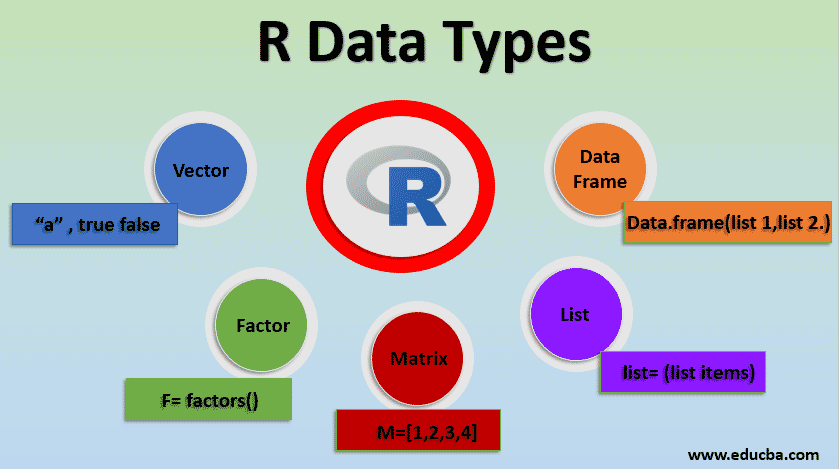## Introduction to R Data Types

R is a programming language helps in carrying out statistical analyses and now has become standard for statistical computing. And comes with some pre-defined functions to do various tasks. Knowledge of Linear algebra will be an added value as it is useful in R and matrix calculations. ‘R’ uses command-line interfaces and accepts commands to work on it by using a prompt >. Let us understand the R Data Types.

### Explain R Data Types

R programming supports various datatypes like scalars, matrices, lists, Vector and data frames. Everything in R is considered to be an object, means it stores and process operations on objects). The key feature of R is a different process is done with different types of objects. Most of the commands in R involves applying functions to the objects. Variables do not require a declaration, instead of assigning a sequence of numbers to the vectors can be done.

Let’s learn the types one by one:

#### 1. Vector

Vector has a set of values with the same types (collection of ordered elements) represented in one dimensional. The class of the vector is determined by the type of the entries made. When a vector is created for more than one element, c () function is used to concatenate all the elements together in a single vector. Vectors are a string of numeric, sequential numbers or random numbers. Vector varieties are the character, integer, numeric, complex, logical (true, false). These are implicit conversion. Some of the functions fives vector functions are  length(), class(x), is.logical(x) , is.null , rep().

Example

In the below, we can see basic vector examples:

• Vector Arithmetic: Numeric vectors are performed in arithmetic expressions to do calculations to give another vector. Statistical operations also are done which gives entries like max, min, var mean.

Code:

```>y >y +2```

Output: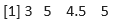The above statement gives the Output by using c() function which adds variable t to 2.

• The length of the vector is calculated by the len () function.

Code:

`> len (y)`

Output: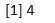• Logical Vectors: Comparison of two numbers with logical values like True, false, NA. Logical operators to satisfy certain conditions include , >=, ==, != for inequality.

Example 1

Code:

```> v > l 0 > l```

Output: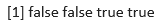Example 2

Code:

```>x=c (3,6,1,2) >x>2```

Output: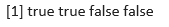Code:

```rep () – to create  replicate values. rep(1,3) rep( 3:6 ,2) rep( 1:3 , each =2) rep(1:3 , times=2 ,each =2)```

• Create a Vector

Code:

```color print (color)```

• To display the class of the vector

Code:

`print ((class (color))`

Output: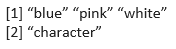In the above program  this one denotes the first element of the vector.

#### 2. Factor

The factor adds numeric codes along with the character level. In simple it defines categorical data with ordered and unordered sets.  They are defined using function factor (). Storing data in a factor helps to store data efficiently in statistical modeling.

Example 1

Code:

```>f = factor (c(1, 6,2,4,7,1,6,7,8) > print (f)```

Output: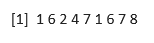Example 2

Code:

```> k = factor (c( 2,0,2,0,0,0 ) , levels =c(0,2) , labels =c( “ prince “ ,”princess”)) >k```

Output: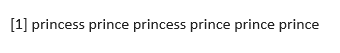#### 3. Matrix

In R programming matrix is a two-dimensional element with numeric and character vectors, simply an atomic vector with the number of rows and columns. Three ways to create a matrix are by using the function matrix(), conversion of the vector into the matrix and binding vectors. Some functions useful here are :

• rbind() and cbind(): combines or binds columns and rows.
• dim(): setting dimensions.

Syntax:

`variable `

Here if it is true it splits by row, false returns split by columns.

Example 1

• Consider a matrix.

Code:

```>x = matrix(c (1,2,3,4,5,6,7,8) 2,4, true) > print (x)```

Output: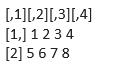Example 2

• Considering Bind.

Code:

```a b cbind( a,b) a   b```

Output: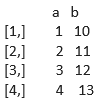#### 4. List

List stores Objects and the elements can be a character, matrices, arrays, numeric. it may consist of another list as an item too.

Syntax:

`variable `

Example of an R list:

Code:

```>lak = list (23, “hi”, cos, list (5L,” l”)) >print (lak)```

Output: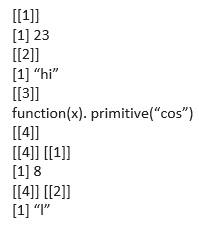Example Considering copies of three Vectors:

Code:

```>a =c(3,5,6) > b =c(“aa”,”cc”,”ee”) > x=c (true, false, true) > y=list(a,b,x)```

Therefore y holds the copies of a,b,x.

#### 5. Data Frame

Data frames are two dimensional with a group of vectors by an equal length. It’s a special kind of list with a rectangular format list. The key factor is to store data tables. They are created using a function data.  the frame ().

Syntax:

`variable `

Example 1

Let’s see an example of the data frame In R.

Code:

```>X= data.frame( values =c(20,50,10), name =c(‘ Gri’,’Tom’,’jeff’)) > print(X) values Name```

Output: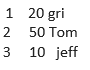Even we can use built-in data frames. In which top element defines a header, followed by data rows and columns. To see the preview, we can use the head function before.

Example 2

Code:

``` >computer Date  intel    speed   data hp   1990    8081   MHZ    8 acer    2001    80286 Mhz     16```

To define the class of the intel:

`>computer [[‘intel’]]`

Output: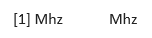### Conclusion

In this article, we have gone through different R data types that are used in programming. To do any application we need variables to store the values and all these variables are necessary to assign data types.  These data types are used in data analysis. Understanding data types help while debugging for computational purposes.

### Recommended Articles

This is a guide to R Data Types. Here we discuss different types in R data with various examples to assign data types. You can also go through our other related articles to learn more –

1. R Data Frame
2. Types of Data Analysis Techniques
3. Best Data Science Programs
4. Types of Data Visualization

The post R Data Types appeared first on EDUCBA.

This post first appeared on Best Online Training & Video Courses | EduCBA, please read the originial post: here